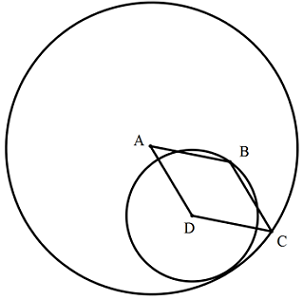# Rhombus

Geometry Level 3A rhombus $ABCD$ has sides of length 10. A circle with center $A$ passes through $C$ (the opposite vertex.) Likewise, a circle with center $D$ passes through $B$. If the two circles are tangent to each other, what is the area of the rhombus?

×